# Kindergarten Subtraction Worksheets

## Free subtraction worksheets

These preschool and kindergarten worksheets help students build basic subtraction skills.  The emphasis initially is on subtraction using objects or pictures to help students understand the meaning of subtraction.  All worksheets are pdf documents.

## Count objects and subtract (within 10)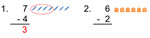These count and subtract worksheets help students understand the connection between counting down and subtracting.

## Count the objects and  write the subtraction equation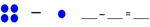In these subtraction with pictures worksheets students are given a subtraction equation in pictures and translate it into an equation.

## Add or subtract 1s or 2s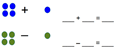In these add and subtract worksheets students add (or subtract) 1 or 2 to or from a number less than 10.

## Subtract (within 5)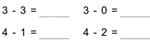Each of these subtract within 5 worksheets provides subtraction questions (no pictures) with all numbers less than 5.

## Subtract the single digit numbers (up to 10, 20)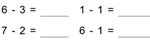We have 18 subtraction worksheets categorized as all numbers within 10within 20 (no borrowing) and within 20 (some borrowing).

## Subtract vertically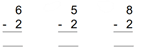These vertical subtraction worksheets provide subtraction problems using the vertical form categorized as all numbers within 10,  within 20 (no borrowing) and within 20 (some borrowing).

## Kindergarten subtraction word problems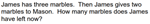Our kindergarten word problem worksheets provide simple word problems which can be solved by subtracting single digit numbers.

## More subtraction worksheets

Explore all of our subtraction worksheets, from subtracting by counting objects to subtracting large numbers in columns.

## What is K5?

K5 Learning offers reading and math worksheets, workbooks and an online reading and math program for kids in kindergarten to grade 5.  We help your children build good study habits and excel in school.